# How to Write your own Flight Controller Software — Part 2

Adding some necessary hardware…

# A Diversion down Hardware Lane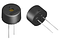Figure 1. 3–30V Passive Piezo.

# The Buzzer Issue

We have selected the PS1440P02BT Piezo (Figure 1) as our noise maker. The data sheet doesn’t provide the current rating for the piezo, possibly because it varies with the voltage used. For 3.3V, the typical maximum current drawn for these devices is 9–14 mA, which is too much for our Nano. Thus we need to drive it via a transistor. Our buzzer driver circuit is shown in Figure 2.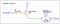Figure 2. Piezo Driver Circuit.

The piezo acts a bit like a capacitor, so we need to place a 1K resistor in parallel with it, to allow it to discharge. Without this, the volume is barely audible at 3.3V. The transistor is a common PNP BC327, which we are using as a switch. When the base emitter voltage, VBE > 0.6V the transistor turns on (saturation), and when VBE < 0.6V the transistor turns off (cutoff). In our schematic, pin 1 is the emitter and pin 2 is the base, thus VE = 3.3V.

This transistor can handle up to 800 mA of collector current (pin 3), which is heaps for our purposes. We can check this by doing some simple calculations.

If the piezo draws a worst case of 14mA, it has an equivalent DC resistance of:

`RP = 3.3 / 0.014 = 236 Ω`

Our total transistor resistance (RL) is RP in parallel with R8 = 191 ohms. Thus our collector current will be:

`IC = VCC / RL = 3.3 / 191 = 17.3 mA (i.e. no problem).`

To work out the base resistor, we can use the following approximation.

`R7 = (VCC — VBE) / (IC / 10)   = (3.3–0.7) / (0.0173 / 10)   = 1503 Ω`

So a 1.5k resistor will work. As we are using the transistor as a switch, there is no harm in pumping a bit more base current than we need to ensure sufficient collector current, so we went with a 1k resistor.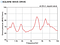Figure 3. Piezo volume vs frequency.

As shown in Figure 3, the volume varies with the frequency. The maximum sound pressure level is around 4 kHz. In the test sketch that we put together, operating near the resonant frequencies makes a significant difference to the volume.

# Software to Drive the Piezo

It took me a while to track down this issue as it isn’t well documented and in fact the Arduino site states that any digital pin can be used for PWM. This may be the case, but only four pins at a time. The Mbed PWM function is where the limitation is arising from, so you could get around this by writing your own PWM function.

To get around this limitation we wrote a function which emulates PWM using bit banging. It is not as good as PWM at higher frequencies but it gets the job done.

UPDATE — While trying to debug the issues we were having decoding SBUS (described in the next section), we came across the Tone class. This uses timers and has a nicer soundthan our version, so we will go with that.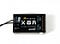Figure 4. FrSKY X8R Remote Control Receiver.

# The SBUS Protocol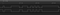Figure 5. A portion of an SBUS packet (inverted and un-inverted).

The SBUS protocol was developed by Futaba to control servos with each channel assigned to a different servo. It is based on RS232 but to make life difficult they inverted the signals (i.e. 0 = VCC and 1 = GND), put the most significant bit first (UART has LSB) and used a non-standard baud rate of 100,000. The other configuration details are (8E2):

• 1 start bit
• 8 data bits
• 1 even parity bit
• 2 stop bits

Each SBUS message is 25 bytes long and takes 3 ms to be transmitted. A Futaba transceiver in analogue mode will send a message every 14 ms, and in high speed mode, every 7 ms. The FrSKY transceivers generally send a message every 10 ms. A message consists of:

• A header byte (0x0F);
• 16 x 11 bit channels (total of 22 bytes);
• 1 flag byte, which includes two digital channels [0 0 0 0 fail_safe frame_lost ch18 ch17]; and
• 1 footer byte (0x00).

Each of the 16 channels can have a value in the range of 172–1811, which we will map to 1000–2000.

We are using the FrSKY X8R Remote Control Receiver (Figure 4) which provides 8 channels using PWM or 16 channels via SBUS. It also gives us signal strength via the RSSI pin and we can transmit telemetry over the Smart Port connection. FrSKY SBUS is inverted compared to Futaba SBUS.

# Binding the Receiver to the Transmitter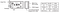Figure 6. FrSky X8R Transmitter Details.

The steps below are the binding procedure for a FrSky X8R receiver. If you are using a different receiver then the binding procedure will be different (read the manual).

1. IGNORE the instructions in the X8R manual — they are confusing!!
2. Disconnect the battery from the drone.
3. As shown in Figure 7, insert the jumpers across the signal pins of channels 1 & 2 and channels 3 & 4. This will configure the receiver as mode 4 (Figure 8). The Flight Controller will use SBUS on CH1-CH16 and the 8 PWM outputs exposed on the X8R will be controlled using CH9-CH16 (which we aren’t using at the moment). The different modes of the X8R are set by a combination of the pins with jumpers and whether the F/S button is pressed while powering on. Mode 4 also provides telemetry which is what we want. Alternatively, leave the jumpers off to get mode 5, which will also work for how we want to use the receiver.
4. Power on the Taranis Transmitter and edit the flight model. Select mode D16 and the number of channels that you want to use. The mode names are a bit misleading, D8 refers to the older D series transmitters with up to 8 channels and telemetry, but without Model Match and Failsafe. These features are only available with D16, which offers a maximum of 16 channels. There is no reason for using D8, except if you are using an older transmitter which does not support D16. Otherwise, D16 is the way to go, even if you only transmit 8 channels.
5. The transmitter and the receiver need to be at least 1 m apart or you may get transmission errors. When you are ready, press bind in the transmitter setup menu.
6. Power up the X8R while holding the F/S button on the module (Figure 4 — if you want mode 4). Release the button. The RED LED on the X8R module will flash, indicating the transmitter is ready to bind to the receiver.
7. Turn off both the transmitter and the receiver. Remove the jumpers on the X8R
8. Turn on the transmitter and connect the battery. The GREEN LED on the receiver indicates the receiver is receiving commands from the transmitter. The receiver/transmitter module binding will not have to be repeated, unless one of the two is replaced.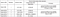Figure 7. Binding Table from the X8R Manual.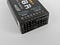Figure 8. Insert jumpers across the signal pins for channels 1 and 2 and 3 and 4.

# Inverting SBUS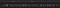Figure 9. FrSKY SBUS Packet.

We need to invert this signal to get a Futaba compatible SBUS packet. In addition, the FrSKY X8R is generating 5V logic and our Nano pins are not 5V tolerant. Consequently, we need to shift the voltage down to 3.3V before feeding the inverted signal to the Nano (Figure 10).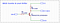Figure 10. SBUS Inverter and Level Shifting Circuit.

The output of this circuit is shown in Figure 5. The top channel of the protocol analyser is showing the SBUS signal straight out of the FrSKY X8R and the bottom channel is showing the inverted signal from the transistor (INV SBUS in Figure 10). This looks perfect because the protocol analyser is cleaning up the signal and showing a purely digital result. We need to have a look on the oscilloscope to see what is really happening.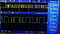Figure 11. FrSKY SBUS on the Oscilloscope.

Figure 11 illustrates the direct FrSKY output on the scope. It isn’t too noisy and the transistions are fairly sharp. Interestingly, even though the X8R is powered from 5V, it seems to be outputting logic at 3.3V (ish). Things are a bit more ropey when we look at the inverted output from the transistor (Figure 12).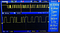Figure 12. Inverted SBUS from Transistor (correct for Futaba SBUS).

In Figure 12 we see that the logic transistions are not quite as crisp as the protocol analyser would have us believe. The rise time has gone from 1 µs to 4 µs, in the next section we will determine whether this matters.

# Arduino SBUS Library

We tried the easy option first, since it looked like it should work. We just added the appropriate board definition in the SBUS::begin() function, as follows:

`#elif defined(ARDUINO_ARCH_NRF52840) // Arduino Nano 33 BLE    _bus->begin(_sbusBaud, SERIAL_8E2);`

We have connected SBUS from the X8R (inverted) to the Rx pin on Serial1 of the Nano 33 BLE. The code above effectively calls Serial1.begin(100000, SERIAL_8E2), thereby setting the baud rate to 100000 and the configuration to 8 data bits, even parity and two stop bits. There are two problems:

1. Setting a non-standard baud rate will be rounded down to the next lowest standard rate (see Figure 12); and
2. The default serial configuration is 8 data bits, no parity, and one stop bit (8N1). Trying to set anything else will cause Mbed OS to crash. This is a known issue.
`if (baudrate < 2400) {new_rate = NRF_UARTE_BAUDRATE_1200;} else if (baudrate < 4800) {new_rate = NRF_UARTE_BAUDRATE_2400;} else if (baudrate < 9600) {new_rate = NRF_UARTE_BAUDRATE_4800;} else if (baudrate < 14400) {new_rate = NRF_UARTE_BAUDRATE_9600…         Figure 12. Extract from serial_api.c (reference).`

It is possible to set a non-standard baud rate and configuration by accessing the appropriate registers for the nRF52840. For example, to set the configuration:

• UART0 Base address 0x40002000
• UART CONFIG register address offset 0x56C
• Therefore register address we want to modify is 0x4000256C

From page 479 of the Product Secification, we want to set:

• STOP = 1 // Two stop bits; and
• PARITY = 0x7 // Include even parity bit

So we want CONFIG = 0x0000000F. For the baud rate (reference):

• UART0 Base address 0x40002000
• UART Baudrate register address offset 0x524

Baudrate formular according Nordic devzone:

• Baudrate = desired baudrate * 2³² / 16000000

The relevant updates to the SBUS library are as follows:

`#if defined(ARDUINO_ARCH_NRF52840) // Arduino Nano 33 BLE    // Serial1.begin() wont set a non-standard baud rate and     // anything but 8N1 crashes Mbed OS.    const uint32_t CONFIG = 0x000000F;    const uint32_t sbusBaudRate = 0x19114A7;    uint32_t *baudRateRegister = ( uint32_t * )0x40002524;    uint32_t *configRegister = ( uint32_t * )0x4000256C;#endif/* starts the serial communication */void SBUS::begin(){    // initialize parsing state    _parserState = 0;    // initialize default scale factors and biases    for (uint8_t i = 0; i < _numChannels; i++){        setEndPoints(i,_defaultMin,_defaultMax);    }       // begin the serial port for SBUS   #if defined(ARDUINO_ARCH_NRF52840) // Arduino Nano 33 BLE      _bus->begin(9600);      *baudRateRegister = sbusBaudRate;      *configRegister = CONFIG;   #else      #error unsupported device   #endif}`

The SBUS “channels” variable in this library is a zero-based array, so channels is actually SBUS channel 1, and so on. The default channel layout on the Taranis Q X7 controller is TAER, and this is what we will use:

`T = Throttle (channel 1);A = Aileron (channel 2);E = Elevator (channel 3); andR = Rudder (channel 4).`

# Monitoring Battery Voltage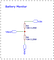Figure 13. Battery Voltage Divider Schematic.

The analog inputs on the Nano 33 BLE can handle a maximum of 3.3V, thus we need a voltage divider to reduce our battery voltage to an acceptable level. Figure 13 illustrates the voltage divider circuit provided on the Magpie Power Distribution Board. The resistor values are appropriate for a 2S LiPo. If you are using something different then you will need to adjust this. The calculation is as follows:

`Fully Charged 2S LiPo = 8.4 VMax Pin Voltage = 3.3 VVbat = Vin x R4 / (R3 + R4)`

where:

`Vbat is the input to our analogue pin; andVin is our battery voltage.`

If we assign a nominal 10k to R4 then R3 = 15.5k, which we round up to 18k for a safety margin and to get a standard value. For our calculation of Vbat to be accurate, we need these resistor values to be accurate so use 1% resistors for R3 and R4. You can use this online voltage divider calculator to play around with different values.

If we rearrange the equation above, we get:

`Vin = Vbat(R3 + R4) / R4 = Vbat * 2.8`

We can use this to calculate the current battery voltage but first we need to convert the analog input value read into a voltage.

The Arduino Nano 33 BLE has 8 analog input pins (ADC 12 bit, 200 ksamples). We have assigned pin A0 (nRF52840 pin PA02, ADC0) to measure our battery voltage and will use the Mbed function AnalogIn.

AnalogIn() reads the voltage as a fraction of the system voltage. The value is a floating point number from 0.0(VSS) to 1.0(VCC). For example, if you have a 3.3V system and the applied voltage is 1.65V, then AnalogIn() reads 0.5 as the value.

The resolution for an ADC is the smallest distinguishable change in analog input that causes the digital output to change. For example, a 12-bit ADC in a 3.3V system has 4,096 distinguishable outputs. Therefore, the resolution of a 12-bit ADC is 3.3/4096 = 0.81mV. This is more accuracy than we need.

Rather than do the calculation, there is another Mbed function which returns the value as a voltage, read_voltage()which returns a float. This is what we will use.

There is a diode between Vusb and Vin to stop a reverse voltage into Vusb from Vin. When Vin is larger than Vusb the board is powered by Vin and Vusb is disconnected. You can test out our example sketch called battery voltage by just connecting the Nano via USB and you will get close to 4.9V (which sounds correct). If both the USB and the battery is connected we read 8.45 V, which is correct for a fully charged 2S LiPo.

# Stay Tuned…

Reefwing Software · iOS & Android Development · Robotics · #followback #iOS #Android #developer #indiedev #robotics #startup #arduino #raspberrypi

## More from David Such

Reefwing Software · iOS & Android Development · Robotics · #followback #iOS #Android #developer #indiedev #robotics #startup #arduino #raspberrypi